# MCQs in Field Effect Transistor Devices

(Last Updated On: December 8, 2017)

This is the Multiple Choice Questions in Field Effect Transistor Devices from the book Electronic Devices and Circuit Theory 10th Edition by Robert L. Boylestad. If you are looking for a reviewer in Electronics Engineering this will definitely help. I can assure you that this will be a great help in reviewing the book in preparation for your Board Exam. Make sure to familiarize each and every questions to increase the chance of passing the ECE Board Exam.

### Online Questions and Answers Topic Outline

• MCQs in FET Devices
• MCQs in Construction and Characteristics of JFETs
• MCQs in Transfer Characteristics
• MCQs in Specification Sheets (JFETs)
• MCQs in Instrumentation
• MCQs in Important Relationships
• MCQs in Depletion-Type MOSFET
• MCQs in Enhancement-Type MOSFET
• MCQs in MOSFET Handling
• MCQs in VMOS
• MCQs in CMOS

### Practice Exam Test Questions

Choose the letter of the best answer in each questions.

1. Which of the following controls the level of ID?

• A) VGS
• B) VDS
• C) IG
• D) VDG

2. Which of the following is (are) not an FET?

• A) n-channel
• B) p-channel
• C) p-n channel
• D) n-channel and p-channel

3. What is the range of an FET’s input impedance?

• A) 10 Ω to 1 kΩ
• B) 1 kΩ to 10 kΩ
• C) 50 kΩ to 100 kΩ
• D) 1 MΩ to several hundred MΩ
4. Which of the following transistor(s) has (have) depletion and enhancement types?
• A) BJT
• B) JFET
• C) MOSFET
• D) None of the above
5. A BJT is a _____-controlled device. The JFET is a _____ – controlled device.
• A) voltage, voltage
• B) voltage, current
• C) current, voltage
• D) current, current

6. The BJT is a _____ device. The FET is a _____ device.

• A) bipolar, bipolar
• B) bipolar, unipolar
• C) unipolar, bipolar
• D) unipolar, unipolar
7. Which of the following is (are) the terminal(s) of a field-effect transistor (FET).
• A) Drain
• B) Gate
• C) Source
• D) All of the above

8. What is the level of IG in an FET?

• A) Zero amperes
• B) Equal to ID
• C) Depends on VDS
• D) Undefined
9. At which of the following is the level of VDS equal to the pinch-off voltage?
• A) When ID becomes equal to IDSS
• B) When VGS is zero volts
• C) IG is zero
• D) All of the above
10. At which of the following condition(s) is the depletion region uniform?
• A) No bias
• B) VDS > 0 V
• C) VDS = VP
• D) None of the above
11. Refer to the following characteristic curve. Calculate the resistance of the FET at VGS = –0.25 V if ro = 10 kΩ.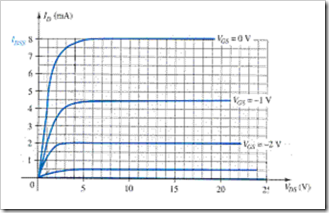• A) 1.1378 kΩ
• B) 113.78 Ω
• C) 11.378 Ω
• D) 11.378 kΩ
12. What is the level of drain current ID for gate-to-source voltages VGS less than (more negative than) the pinch-off level?
• A) zero amperes
• B) IDSS
• C) Negative value
• D) Undefined

13. The three terminals of the JFET are the _____, _____, and _____.

• A) gate, collector, emitter
• B) base, collector, emitter
• C) gate, drain, source
• D) gate, drain, emitter
14. The level of VGS that results in ID = 0 mA is defined by VGS = _____.
• A) VGS(off)
• B) VP
• C) VDS
• D) None of the above
15. The region to the left of the pinch-off locus is referred to as the _____ region.
• A) saturation
• B) cutoff
• C) ohmic
• D) All of the above

16. Which of the following represent(s) the cutoff region for an FET?

• A) ID = 0 mA
• B) VGS = VP
• C) IG = 0
• D) All of the above
17. Referring to this transfer curve. Calculate (using Shockley’s equation) VGS at ID = 4mA.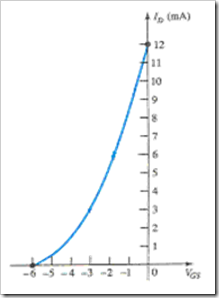• A) 2.54 V
• B) –2.54 V
• C) –12 V
• D) Undefined

18. Referring to this transfer curve, determine ID at VGS = 2 V.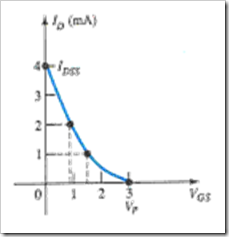• A) 0.444 mA
• B) 1.333 mA
• C) 0.111 mA
• D) 4.444 mA

19. What is the ratio of ID / IDSS for VGS = 0.5 VP?

• A) 0.25
• B) 0.5
• C) 1
• D) 0
20. The drain current will always be one-fourth of IDSS as long as the gate-to-source voltage is _____ the pinch-off value.
• A) one-fourth
• B) one-half
• C) three-fourths
• D) None of the above
21. Which of the following ratings appear(s) in the specification sheet for an FET?
• A) Voltages between specific terminals
• B) Current levels
• C) Power dissipation
• D) All of the above
22. Refer to this portion of a specification sheet. Determine the values of reverse-gate-source voltage and gate current if the FET was forced to accept it.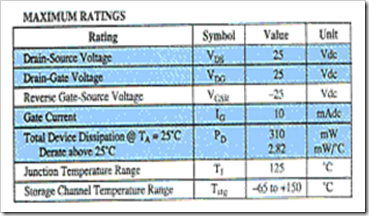• A) 25 Vdc, –200 nAdc
• B) –25 Vdc, 10 mAdc
• C) –6 Vdc, –1.0 nAdc
• D) None of the above

23. Hand-held instruments are available to measure _____ for the BJT.

• A) βdc
• B) IDSS
• C) VP
• D) All of the above

24. How many terminals can a MOSFET have?

• A) 2
• B) 3
• C) 4
• D) 3 or 4

25. Which of the following applies to MOSFETs?

• A) No direct electrical connection between the gate terminal and the channel
• B) Desirable high input impedance
• C) Uses metal for the gate, drain, and source connections
• D) All of the above
26. Referring to the following transfer curve, determine the level of VGS when the drain current is 20 mA.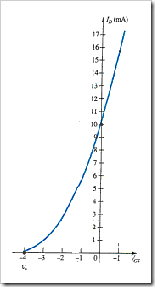• A) 1.66 V
• B) –1.66 V
• C) 0.66 V
• D) –0.66 V

27. Refer to the following curves. Calculate ID at VGS = 1 V.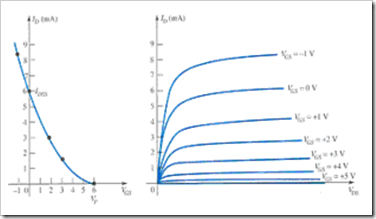• A) 8.167 mA
• B) 4.167 mA
• C) 6.167 mA
• D) 0.616 mA
28. It is the insulating layer of _____ in the MOSFET construction that accounts for the very desirable high input impedance of the device.
• A) SiO
• B) GaAs
• C) SiO2
• D) HCl
29. Refer to the following figure. Calculate VGS at ID = 8 mA for k = 0.278 × 10–2 A/V2.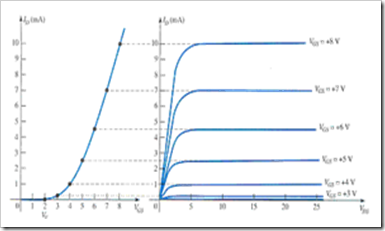• A) 3.70 V
• B) 5.36 V
• C) 7.36 V
• D) 2.36 V
30. The transfer curve is not defined by Shockley’s equation for the _____.
• A) JFET
• B) depletion-type MOSFET
• C) enhancement-type MOSFET
• D) BJT

31. Which of the following applies to a safe MOSFET handling?

• A) Always pick up the transistor by the casing.
• B) Power should always be off when network changes are made.
• C) Always touch ground before handling the device.
• D) All of the above
32. What is the purpose of adding two Zener diodes to the MOSFET in this figure?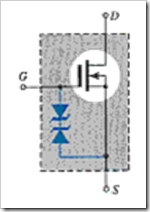• A) To reduce the input impedance
• B) To protect the MOSFET for both polarities
• C) To increase the input impedance
• D) None of the above
33. Which of the following is (are) the advantage(s) of VMOS over MOSFETs?
• A) Reduced channel resistance
• B) Higher current and power ratings
• C) Faster switching time
• D) All of the above

34. Which of the following FETs has the lowest input impedance?

• A) JFET
• B) MOSFET depletion-type
• C) MOSFET enhancement-type
• D) None of the above

35. Which of the following input impedances is not valid for a JFET?

• A) 1010 Ω
• B) 109 Ω
• C) 108 Ω
• D) 1011 Ω

### Fill-in-the-blanks Questions

1. A junction field-effect transistor (JFET) is a _____ device.

• A) current-controlled
• B) voltage-controlled
• C) voltage-current controlled
• D) None of the above
2. The FET is a _____ device depending solely on either electron (n-channel) or hole (p-channel) conduction.
• A) unipolar
• B) bipolar
• C) tripolar
• D) None of the above
3. One of the most important characteristics of the FET is its _____ impedance.
• A) low input
• B) medium input
• C) high input
• D) None of the above
4. The _____ transistor has become one of the most important devices used in the design and construction of integrated circuits for digital computers.
• A) MOSFET
• B) BJT
• C) JFET
• D) None of the above
5. In the n-channel transistor, the drain and source are connected to the _____ channel while the gate is connected to the two layers of _____ material.
• A) p-type, n-type
• B) p-type, p-type
• C) n-type, p-type
• D) n-type, n-type
6. In an FET transistor, the depletion region is _____ near the top of both p-type materials.
• A) wider
• B) narrower
• C) the same as the rest of the depletion region
• D) None of the above
7. The pinch-off voltage continues to drop in a _____ manner as VGS becomes more and more negative.
• A) linear
• B) parabolic
• C) cubic
• D) None of the above
8. The region to the right of the pinch-off locus is commonly referred to as the _____ region.
• A) constant-current
• B) saturation
• C) linear amplification
• D) All of the above
9. As VGS becomes _____ negative, the slope of each curve in the characteristics becomes _____ horizontal corresponding with an increasing resistance level.
• A) less, more
• B) more, less
• C) more, more
• D) None of the above

10. The transfer curve can be obtained by _____.

• A) using Shockley’s equation
• B) using both Shockley’s equation and by output characteristics
• C) characteristics
• D) None of the above

11. The active region of an FET is bounded by _____.

• A) ohmic region
• B) cutoff region
• C) power line
• D) All of the above

12. A(n) _____ can be used to check the condition of an FET.

• A) digital display meter (DDM)
• B) ohmmeter (VOM)
• C) curve tracer
• D) All of the above
13. In a curve tracer, the _____ reveals the distance between the VGS curves for the n-channel device.
• A) vertical sens.
• B) horizontal sens.
• C) Per step
• D) gm
14. In an FET circuit, _____ is normally the parameter to be determined first.
• A) VGS
• B) VDS
• C) VDG
• D) ID
15. The primary difference between the construction of a MOSFET and an FET is the _____.
• A) construction of the gate connection
• B) low input impedance
• C) threshold voltage
• D) None of the above
16. The primary difference between the construction of depletion-type and enhancement-type MOSFETs is _____.
• A) the size of the transistor
• B) the absence of the channel
• C) the reverse bias junction
• D) All of the above
17. The level of _____ that results in the significant increase in drain current in enhancement-type MOSFETs is called threshold voltage VT’.
• A) VDD
• B) VDS
• C) VGS
• D) VDG
18. In an n-channel enhancement-type MOSFET with a fixed value of VT’, the _____ the level of VGS’, the _____ the saturation level for VDS’.
• A) higher, more
• B) higher, less
• C) lower, lower
• D) None of the above

19. The enhancement-type MOSFET is in the cutoff region if _____.

• A) applied VGS is larger than VGS(Th)
• B) applied VGS is less than or equal to VGS(Th)
• C) VGS has a positive level
• D) None of the above
20. The specification sheet provides _____ to calculate the value of k for enhancement-type MOSFETs.
• A) VGS(on)
• B) ID(on)
• C) VGS(Th)
• D) All of the above
21. _____ has high input impedance, fast switching speeds, and lower operating power levels.
• A) CMOS
• B) FET
• C) BJT
• D) None of the above
22. The FET resistance in the ohmic region is _____ at VP and _____ at the origin.
• A) smallest, largest
• B) largest, smallest
• C) larger, smaller
• D) smaller, larger

23. The silicon dioxide (SiO2) layer used in a MOSFET is _____.

• A) an insulator
• B) a conductor
• C) a semiconductor
• D) None of the above
24. In an n-channel depletion-type MOSFET the region of positive gate voltages on the drain or transfer characteristics is referred to as the _____ region with the region between cutoff and the saturation level of ID referred to as the _____ region.
• A) depletion, enhancement
• B) enhancement, enhancement
• C) enhancement, depletion
• D) None of the above

25. VMOS FETs have a _____ temperature coefficient that will combat the possibility of thermal runaway.

• A) positive
• B) negative
• C) zero
• D) None of the above